## Development of the Ideal Gas Law

The pressure, volume, temperature, and amount of an ideal gas are related by one equation that was derived through the experimental work of several individuals, especially Robert Boyle, Jacques A. C. Charles, and Joseph Gay‐Lussac. An ideal gas consists of identical, infinitesimally small particles that only interact occasionally like elastic billiard balls. Real gases act much like ideal gases at the usual temperatures and pressures found on the earth's surface. The gases in the sun are not ideal gases due to the high temperature and pressures found there.

## Boyle's law

If a gas is compressed while keeping the temperature constant, the pressure varies inversely with the volume. Hence, Boyle's law can be stated thus: The product of the pressure (P) and its corresponding volume (V) is a constant. Mathematically, PV = constant. Or, if P is the original pressure, V is the original volume, P′ represents the new pressure, and V′ the new volume, the relationship is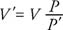## Charles/Gay-Lussac law

The Charles/Gay‐Lussac law denotes that for a constant pressure, the volume of a gas is directly proportional to the Kelvin temperature. In equation form, V = (constant) T. Or if V is the original volume, T the original Kelvin temperature, V′ the new volume, and T′ the new Kelvin temperature, the relationship is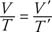Boyle's law and the Charles/Gay‐Lussac law can be combined: PV = (constant) T. The volume increases when the mass (m) of gas increases as, for example, pumping more gas into a tire; therefore, the volume of the gas is also directly related to the mass of the gas and PV = (constant) mT.

## Definition of a mole

The proportionality constant of the previous equation is the same for all gases if the amount of gas is measured in moles rather in terms of mass. The number of moles (n) of gas is the ratio of the mass (m) and the molecular or atomic mass (M) expressed in grams per mole: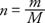The mole of pure substance contains a mass in grams equal to the molecular mass or atomic mass of the substance. For example, lead has an atomic mass of 207 g/mole, or 207 g of lead is 1 mole of lead.

## The ideal gas law

Incorporating Boyle's law, the Charles/Gay‐Lussac law, and the definition of a mole into one expression yields the ideal gas law PV = nRT, where R is the universal gas constant with the value of R = 8.31 J/mole‐degree × K in SI units, where pressure is expressed in N/m 2 (pascals), volume is in cubic meters, and temperature is in degrees Kelvin.

If the temperature, pressure, and volume change for a given number of moles of gas, the formula is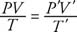where unprimed variables refer to one set of conditions and the primed variables refer to another. Frequently, a set of conditions of the temperature, pressure, and volume of a gas are compared to standard temperature and pressure (STP). Standard pressure is 1 atmosphere, and standard temperature is 0 degrees Celsius (approximately 273 degrees Kelvin).

Amadeo Avogadro (1776–1856) stated that one mole of any gas at standard pressure and temperature contains the same number of molecules. The value called Avogadro's number is N = 6.02 × 10 23 molecules/mole. The ideal gas law can be written in terms of Avogadro's number as PV = NkT, where k, called the Boltzmann's constant, has the value k = 1.38 × 10 −23 J/K. One mole of any gas at standard temperature and pressure (STP) occupies a standard volume of 22.4 liters.

## The kinetic theory of gases

Consider a gas with the four following idealized characteristics:

• It is in thermal equilibrium with its container.
• The gas molecules collide elastically with other molecules and the walls of the vessel.
• The molecules are separated by distances that are large compared to their diameters.
• The net velocity of all the gas molecules must be zero so that, on the average, as many molecules are moving in one direction as in another.

This model of a gas as a collection of molecules in constant motion undergoing elastic collisions according to Newtonian laws is the kinetic theory of gases.

From Newtonian mechanics, the pressure on the wall (P) may be derived in terms of the average kinetic energy of the gas molecules:The result shows that the pressure is proportional to the number of molecules per unit volume (N/V) and to the average linear kinetic energy of the molecules. Using this formula and the ideal gas law, the relationship between temperature and average linear kinetic energy can be found: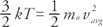where k is again Boltzmann's constant; therefore, the average kinetic energy of gas molecules is directly proportional to the temperature of the gas in degrees Kelvin. Temperature is a direct measure of the average molecular kinetic energy for an ideal gas.

These results seem intuitively defensible. If the temperature rises, the gas molecules move at greater speeds. If the volume remains unchanged, the hotter molecules would be expected to hit the walls more often than cooler ones, resulting in an increase in pressure. These significant relationships link the motions of the gas molecules in the subatomic world to their characteristics observed in the macroscopic world.